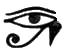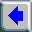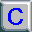## [Printed book in 3 volumes, plus Pdf, Epub, Mobi.]## Recovering the Lost World, A Saturnian Cosmology -- Jno Cook Appendix N: Age of the Universe.\$Revision: 42.11 \$ (age.php)
Contents of this Appendix: [The Milky Way] [The Local Group] [The Virgo Supercluster] [The Visible Universe] [Endnotes]

## The Age of the Universe

The accepted age of the Universe is a modest 15 billion years, based on a "Big Bang" event at the beginning of time. I won't bring forward the accumulated data which argues against the "Big Bang" -- a derisive term coined by Fred Hoyle, which stuck.

## The Milky Way

It would be better to take a look at Halton Arp's book Seeing Red (1998). Our galaxy is the daughter of a nearby galaxy in Virgo, and we in turn seem to be the mother and grandmother of two additional generations of offspring galaxies. I have seen recent estimates that it would take 100 billion years to "form" a galaxy. But Arp suggests (through an example) as little as 8 billion years. I'll use the very conservative value of 8 billion years between generations. As a grandmother, the Milky Way is three generations old.

Age of the Milky Way galaxy
3 times 8 = 24 billion years.

If the Milky Way is typical of a leaf on the branches of the Universe, then the Universe might be very old. If we could count galaxies (they count in the billions), and get some idea of the connections between them (they tend to form in groups), we could form an estimate. If there are indeed billions of galaxies, and they multiply by sending new pairs out from their center (as Arp has suggested) every 8 billion years, then the galaxies have multiplied like rabbits. I'll do the math for a Fibonacci series. [note 1]

## The Local Group

The local group, to which the Milky Way belongs, contains only about 40 galaxies. The 9th term of the Fibonacci series reaches a value of 34. That represents 9 generations, assuming that this portion of the Universe started from a single other galaxy.

Age of the Local Group of galaxies
9 times 8 = 72 billion years.

## The Virgo Supercluster

But we are part of the Virgo Supercluster which contains about 27,500 galaxies. The 23rd term of the Fibonacci series reaches a value of 28,657, representing 23 generations.

Age of the Virgo Supercluster
23 times 8 = 184 billion years.

## The Visible Universe

A web source lists 3,850 billion galaxies in the "visible" Universe. The 62nd Fibonacci term is 4,054 billion.

Age of the Visible Universe
62 times 8 = 496 billion years.

The really big numbers generated by a Fibonacci Series increase much faster than the index of the series. It is the "index" which is a measure of age. Thus there might be a finite number which represents the real age of the Universe. However, there are plenty of people who believe the Universe is infinite in size and infinite in age. [note 2]

## Endnotes

Note 1 --

The estimates on the galaxies at various visual spacings from Earth are from Richard Powell, [www.atlasoftheuniverse.com].

For an expansion of Fibonacci terms see [www.maths.surrey.ac.uk/hosted-sites/R.Knott/Fibonacci/fib.html]

Note 2 --

Lars Wåhlin, in The Deadbeat Universe (1997, rev. 2003), estimates that one fourth of the life of a collapsing universe has passed, which will end at the count of 2.47 * 10^20 seconds. One fourth of this is equal to 1.95 * 10^12 years -- 1,950 billion years. That is about 4 times the value I derived.

Dewey Larson, in The Structure of the Physical Universe (1959), suggests that the Universe is not infinite in size, but only in time, and suggests a replenishment from a parallel time-based Universe.

Calculations are in Unix bc notation, where ^ denotes exponentiation; the functions a(rctangent), s(ine), and c(osine) use radians; angle conversions to radians or degrees by the divisors rad=.017+ and deg=57.2+; other functions are shown as f( ); tan( )=s( )/c( )
units: million == 1,000,000; billion == 1,000,000,000;
AU == 93,000,000 miles.